Courses

# Surveying (Part - 11) Civil Engineering (CE) Notes | EduRev

## Civil Engineering (CE) : Surveying (Part - 11) Civil Engineering (CE) Notes | EduRev

The document Surveying (Part - 11) Civil Engineering (CE) Notes | EduRev is a part of the Civil Engineering (CE) Course Civil Engineering SSC JE (Technical).
All you need of Civil Engineering (CE) at this link: Civil Engineering (CE)

Surveying (Part 11)

Deflection angle (d) and angle of deflection (f) are different things.
In this method the curve is set out by the tangential angle called the deflection angle with a theodolite and a chain or tope.
Derivation of the Formula :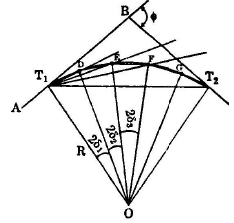Let AB = rear tangent of the curve T1and T2 = tangent points D,E,F etc = successive points on the curve d1,d2,d3
= tangential angles, which each of the successive chords  T1, D, DE, EF etc makes with the respective tangents at T1, D, E, etc.
D1,D2,D3 etc = total tangential or deflection angles (between the rear tangent AB and each of the line T1D, T1E, T1F etc) for the chord T1D, DE, EF etc.
C1, C2, C3 etc. = length of the subchord and normal chord T1D, DE, EF etc.
R = Radius of the curve.
Chord T1D = are T1D � C1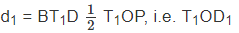now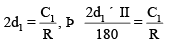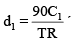60 minutes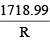minutes

Similarly,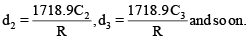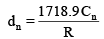Since each of chord lengths C2, C3 ......... Cn-1 is  equal to the length of the unit chord , d2 = d3 = d ....... = dn-1.
Hence the total tengential angle (deflection angle)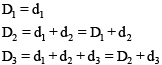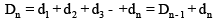Check: total deflection angle (BT1T2) = D

�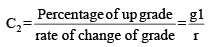�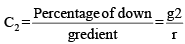Total length of the vertical curves,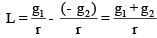� h = kN2 = Tangent correction where k is a constant and N is the distance measured from O.

TRINGULATION
The process of measuring the anges of a chosen networks of triangles formed by a number of station marked on the surface of the earth is called triangulation.
Principle of Triangulation If all the three angles and the length of one its sides of a triangles are known, then the lengths of the remaining sides of the triangle can be calculated trignometrically. 

• The sides of the first triangle, whose length is predetermined is called the base line and vertices of the individual triangles are known as triangulation stations and the whose figure is called the triangulation system or triangulation figure. 
• The triangulation stations at which astronomical observations for azimuth are made are called Laplace stations.

Classification of Triangulations

(1) Primary triangulation of first order triangulation: Highest grade of triangulations.
(2) Secondary triangulation or Second order triangulation
(3) Tertiary triangulation or third order triangulation or Topo triangulation

Specification of primary secondary and Tertiary triangulation

 Item Primary Secondary Tertiary (1) Length of the base links 8 to 12 km 2 to 5 km 100 to 500 km (2) Length of the sides 16 km to 150 km 10 to 25 km 2 to 10 km (3) Averagetriangulation error less than 1" 3" 12" (4) Maximum station closure not more than 3" 8" 15" (5) Actual error of the base 1:50,000 1 in 25000 1 in 10,000 (6) Probable error of the base 1:1000000 1 in 500000 1 in 250000

Phase of a Signal When a cylindrical opaque signal which is partly illuminated by the sun and partly remain in shadow, is observed, the observer sees only illuminated portion from his station and thus bisects the centre of the illuminated portion. The error of bisection thus introduced is known as phase of the signal.

Offer running on EduRev: Apply code STAYHOME200 to get INR 200 off on our premium plan EduRev Infinity!

## Civil Engineering SSC JE (Technical)

109 docs|50 tests

,

,

,

,

,

,

,

,

,

,

,

,

,

,

,

,

,

,

,

,

,

;